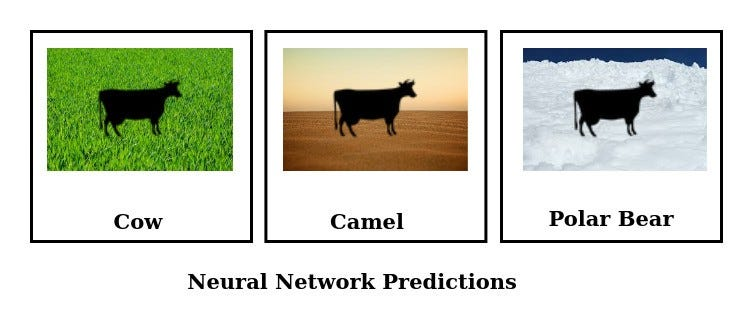# Case Study: Is Spurious Correlations the reason why Neural Networks fail on unseen data?

1. Understand spurious correlations and how they occur in data
2. Understand how and why neural networks fail on new data due to spurious correlations
3. Learn data-centric strategies (Domain Randomization & Data Augmentation) to minimize these failures

## Cow Grass ExampleImage by Author, inspired from Recognition in Terra Incognita

# Data

`Y = x1 + 2*x2 + 3 + N(0,1)X3 = Y + N(0,3)`
• X1 is a causal feature with coefficient=1
• X2 is a casual feature with coefficient=2
• X3 has a strong spurious correlation with y
`# training dataX,y = get_data(n=5000, spurious_correlation_factor=0.2)# iid data with same distribution as trainingiid_X,iid_y = get_data(n=5000, spurious_correlation_factor=0.2)## ood data with a different correlation factor to X3ood_X,ood_y = get_data(n=5000, spurious_correlation_factor=0.1)`

# Model

## Training

`optimizer = torch.optim.Adam(model.parameters(), lr=1e-3, betas=(0.9, 0.999), eps=1e-08)scheduler = torch.optim.lr_scheduler.StepLR(optimizer, step_size=10, gamma=0.5)criterion = nn.MSELoss()NUM_EPOCHS = 30`

# Potential Solutions

1. Domain Randomization: Collect data randomly from many different domains. In analogy to cow-grass example if we collected datasets from different countries where association strength changes, our model will learn to build invariance to grassy background.
2. Use data augmentation to randomize this property. Data augmentations for building invariance are transforms that randomly change a certain property of the dataset without changing the output.

## Conclusions

1. Datasets are biased: spurious correlations can creep into your data due to various reasons and this needs to be dealt with consciously.
2. Neural Networks are prone to data failures: Dataset biases can seriously derail the neural network from intended solution, which can result in spectacular failures when they are applied to a different distribution.
3. OOD Evaluations are necessary: when ever you have access to OOD dataset it is good practice to measure performance on it, since iid performance alone is not a good measure of model credibility.
4. Data Augmentation is your friend: data augmentation has been widely celebrated for ability to create more data from existing data. But as we have seen, what it actually does is to randomize a known non-causal property to ensure that network is invariant to this property.
5. Representational Data: Rather than only focusing on volume we should try to get as much variation in data as possible, so that most non-causal properties are randomized naturally.

## References

Unlisted

--

--## Urwa Muaz

Computer Vision Practitioner |Data Science Graduate, NYU | Interested in Robust Deep Learning